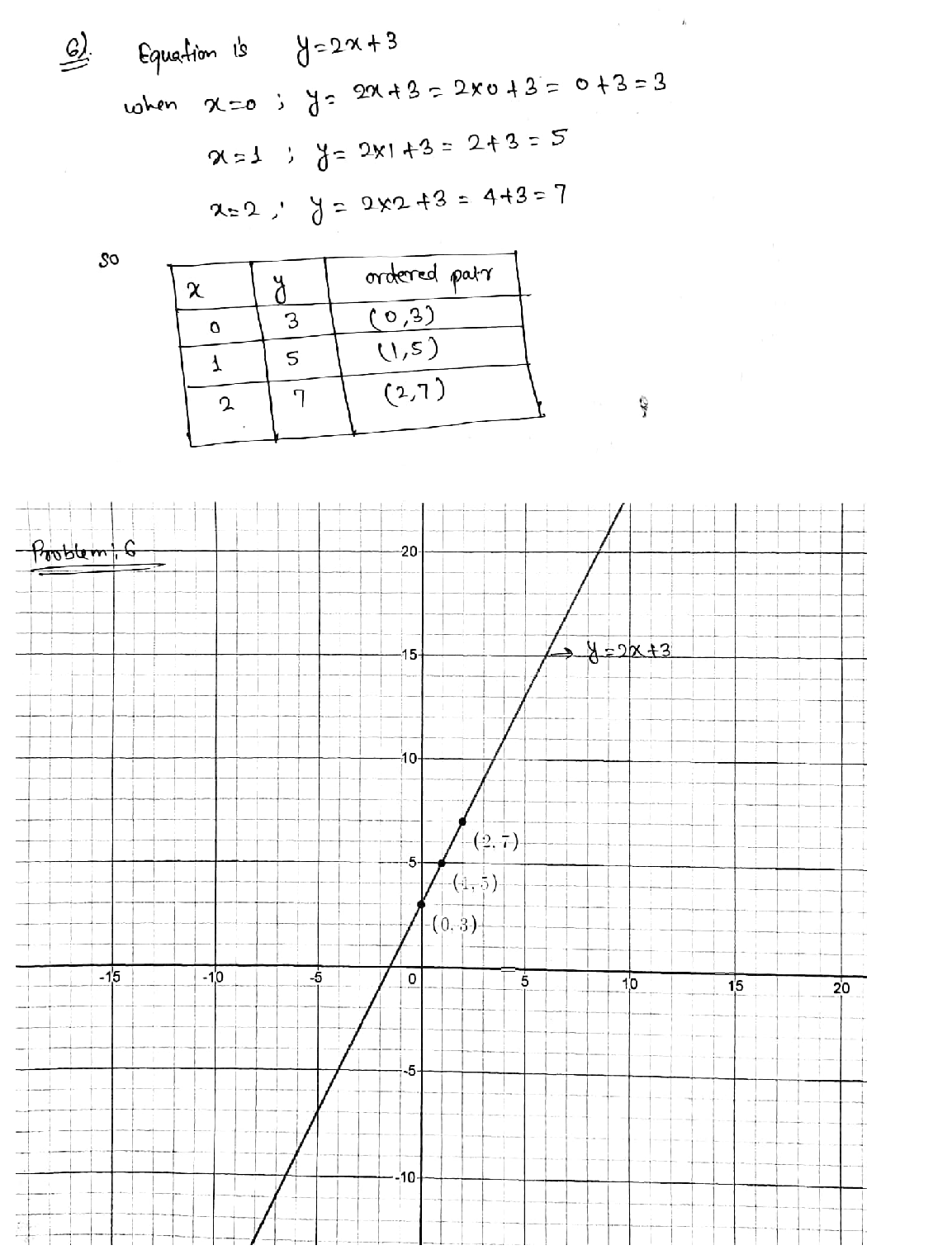Question

Linear Algebra

6. Graph the equation and determine at least 3 solutions for: y=2x +3Verified### Question 45665Linear Algebra

\text { 4] Find } g(0)-g(9)+g(2) \text {, if }
g(x)=\left\{\begin{aligned} \frac{x+1}{2}, & \text { if } x \text { is odd } \\ \frac{x}{2}, & \text { if } x \text { is even } \end{aligned}\right.

### Question 45664Linear Algebra

\text { 3] Find } f(4)-f(2)+f(3) \text {, if }
f(x)=\left\{\begin{array}{ll} \frac{x+1}{2}, & \text { if } x \text { is odd } \\ \frac{x}{4}, & \text { if } x \text { is even } \end{array}\right.

### Question 45663Linear Algebra

2] Find the Domains of the following functions:
\text { a) } f(x)=\sqrt{15-5 x}
f(x)=\frac{x^{2}-2 x+1}{x^{2}-4 x-21}
f(x)=\frac{x^{2}-2 x+1}{\sqrt{16-2 x}}

### Question 45662Linear Algebra

a) Give the definition of a rational function. [5 pts]
b) Give an example of a polynomial function of degree 3. [5 pts]
c) Can a constant function be a polynomial and a rational function at the same time? Explain your answer. [5 pts]
d) Give an example of a non-polynomial function and explain why not apolynomial function. [10 pts)

### Question 45333Linear Algebra

5) Ве,
\begin{array}{c} f: \mathbb{R} \rightarrow \mathbb{R} \\ f(x)=\left\{\begin{array}{ll} x^{2}-3 \cos (\pi x) & x<0 \\ x-4 e^{-2 x} & x \geq 0 . \end{array}\right. \end{array}
Calculate
\int_{-1}^{2} f(x) d x
Presenting the result in simplified form

### Question 45332Linear Algebra

4) Be f: R - Ra function differentiable in R such your derivative f', has in maximum a real zero.
Prove that the equation f(x)=0 has in maximum 2 real square

### Question 45331Linear Algebra

3) Take in consideration the following function
f(x)=\left\{\begin{array}{ll} f: \mathbb{R} \rightarrow \mathbb{R} & \\ \frac{x^{2}-4 x+\cos (\sin (x)),}{x^{4}+4 x^{2}+1}, & x \leq 0 \end{array}\right.
a) Show that the function f is continuous in R+ and in R- but discontinuous in the point X
b) Say justifying, if f is differenciable in X=0

### Question 45330Linear Algebra

2) Prove by definition that
\lim _{x \rightarrow 0} x^{2} \cos \left(e^{x}\right)=0

### Question 45329Linear Algebra

1) Calculate the following limit:
\lim _{x \rightarrow+\infty} \frac{x^{2}\left(e^{-3 x}+1\right)+x \cos (5 x)}{x^{2}+7 x+1}

### Question 45305Linear Algebra

7. Consider the following problem.
Maximize Z = 6X1 + 8X2
5X1 + 2X2 <=20
X1 + 2X2 <=10
X1, X2 >= 0
a. Construct the dual problem for this primal problem
b. Solve both the primal problem and the dual problem graphically.Identify the CPF (Corner-Point Feasible) solutions and corner -pointinfeasible solutions for both problems. Calculate the objectivefunction values for all these solutions.
c. Construct a table listing the complementary basic solutions for theseproblems.
d. Work through the simplex method step by step to solve the primal problem. After each iteration, identify the basic feasible (BF) solution for this problem and the complementary basic solution for the dual problem. Also identify the corresponding corner-point solutions.

### Submit query